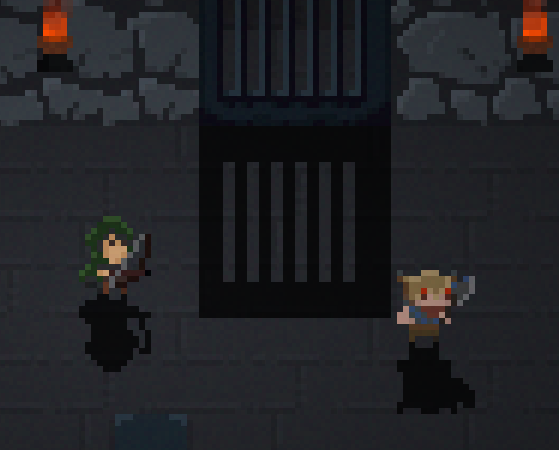# 2D Sprite Reflections

First post in a year! So much for me writing more regularly on this blog I guess… on a more positive note, I have been making steady progress on a game. The concept has shifted quite a bit from where I started off last year, but I guess that was to be expected during the initial phase. Nonetheless, my ideas for concept & mechanics have solidified quite a bit, and I’m starting to feel like I may actually have something playable to present here in the not-so-distant future.

But that’s enough of these reflections for now – let’s look at into something more fun. I wanted to add the ability to have reflecting surfaces in my game world that could be used for mirrors, shiny surfaces, or water. The game engine renders all objects as 2D sprites which are arranged on different layers. For example, there is “floor” layer which holds the ground surface, a “scene” layer that contains the player and enemies, and so on. Each of these layers can have effects attached to it that can change the way these sprites are rendered, as well as a shader that determines how the layer is added to generate the final image on screen.

Here’s the original effect shader used to composite layers on top of each other:

```in vec2 v_tex_coord;
uniform sampler2D u_tex0;
out vec4 o_color;

void main()
{
// Sample original sprite
vec4 original = texture(u_tex0, v_tex_coord);
o_color = original;
}
```

And here’s an example of what a character sprite would look like: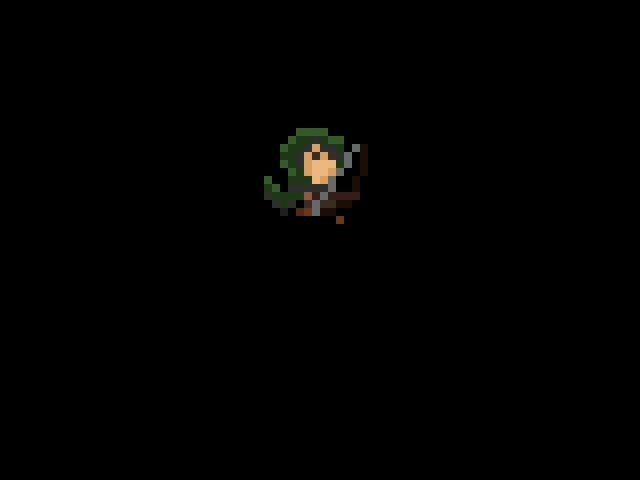My initial idea was to create an composite shader that would mirror the whole layer at once to create the reflection. For that I needed to flip the y-coordinate in the fragment shader, so that the effect renders a copy of the original sprite that is flipped vertically in addition to the original sprite:

```void main()
{
// Sample original sprite
vec4 original = texture(u_tex0, v_tex_coord);
// Sample once more for mirrored location
vec4 mirror = texture(u_tex0, vec2(v_tex_coord.x, 1.0 - v_tex_coord.y));
// output final color from original or mirrored sample
o_color = original + mirror;
}
```

That shader produced a mirrored copy: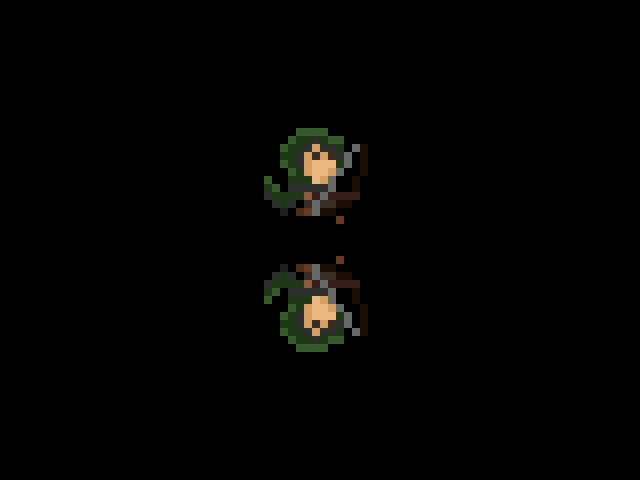Note that this works here because we’re writing onto an otherwise empty layer, meaning that the sprite doesn’t overlap with any other sprites.

Next, I tweaked the shader slightly to reduce the reflectivity to make the mirrored version of the sprite stand out less. In general, the reflectivity factor corresponds to how much light a surface reflects and can be used to model different surface materials.

```void main()
{
vec4 original = texture(u_tex0, v_tex_coord);;
vec4 mirror = texture(u_tex0, vec2(v_tex_coord.x, 1.0 - v_tex_coord.y));
// multiply reflectivity into mirrored sprite
float reflectivity = 0.7;
o_color = reflectivity * mirror + original;
}
```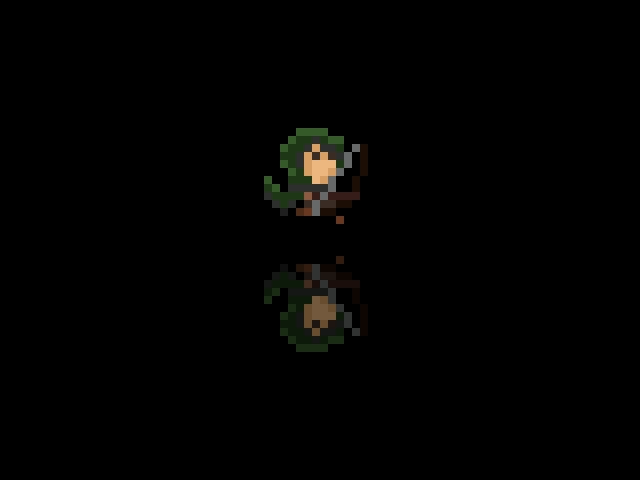As I was moving the character around the screen, I noticed a problem: in my simple example, I had made the assumption that I could just invert the y-coordinate so that for example, y=0 turned into y=1, and vice versa. Effectively, I was using the middle of the screen as my axis of reflection. Here’s what happened once I moved the sprite along the y-axis (up / down):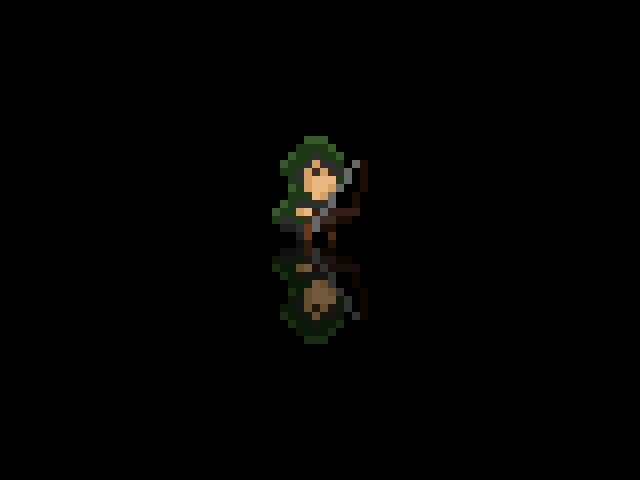As you can see, regardless of where on the screen the sprite is, the reflected image is always relative to the distance from the middle / axis. Instead, that meant that each sprite in the game world would need to have its own axis of reflection depending on it’s y-position. Thus, a single reflection pass would not work.

My next idea was to render each sprite twice: once, as part of the reflection pass to render the mirrored version, and then as before to render the actual character. During the reflection pass, I would need to shift the sprite down and flip it along it’s horizontal axis as before. Here’s the original vertex shader used to render a single sprite:

```void main()
{
// position on screen
gl_Position = u_cam.proj_orth * u_cam.view * u_model * vec4(a_position, 0.0, 1.0);
// texture coordinates in sprite map
v_tex_coord = u_uvwh.xy + u_uvwh.zw * a_tex_coord;
}
```

This was changed to shift the reflection below the original:

```void main()
{
// Shift y position by height of the sprite
vec4 pos = vec4(a_position.x, a_position.y + 1, 0.0, 1.0);
gl_Position = u_cam.proj_orth * u_cam.view * u_model * pos;
v_tex_coord = u_uvwh.xy + u_uvwh.zw * a_tex_coord;
}
```

With these changes, the sprite now correctly reflects anywhere on screen using the lower border of the sprite as the reflection axis: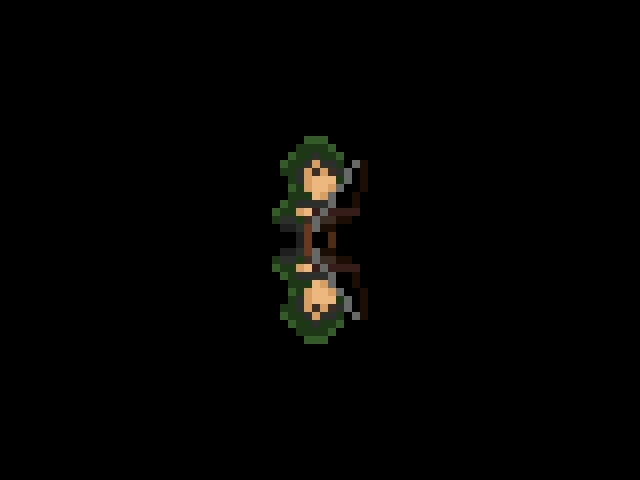Next, I wanted to add back in the reflectivity factor that I described earlier. Here’s the original fragment shader to render a single sprite:

```void main()
{
vec4 material = texture(u_tex0, v_tex_coord);
o_color = u_color * material;
}
```

And here’s the same shader with the reflectivity factor included:

```void main()
{
vec4 material = texture(u_tex0, v_tex_coord);
float reflectivity = 0.7;
o_color = reflectivity * u_color * material;
}
```

So far, so good: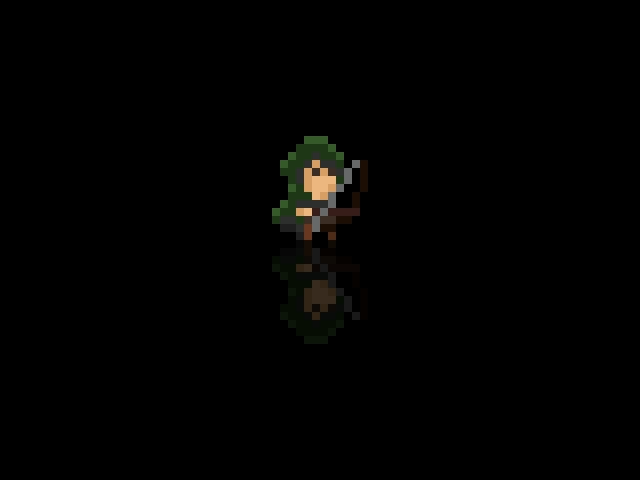However, I wanted to have more control over which areas of the screen are reflective. In my game, this will allow me to have locally limited reflectitions. Instead of using a constant reflectiveness, I decided to use a 1-channel mask that indicates how reflective any given pixel on screen will be. Here’s the final fragment shader:

```void main()
{
vec4 original = texture(u_tex0, v_tex_coord);
// sample reflectivity from map
float reflectivity = texture(u_tex1, v_tex_coord).r;
o_color = reflectivity * original;
}
```

Here’s an example of a circular reflection map that allows for soft transitions: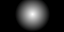Notice how the reflection fades out around the edges: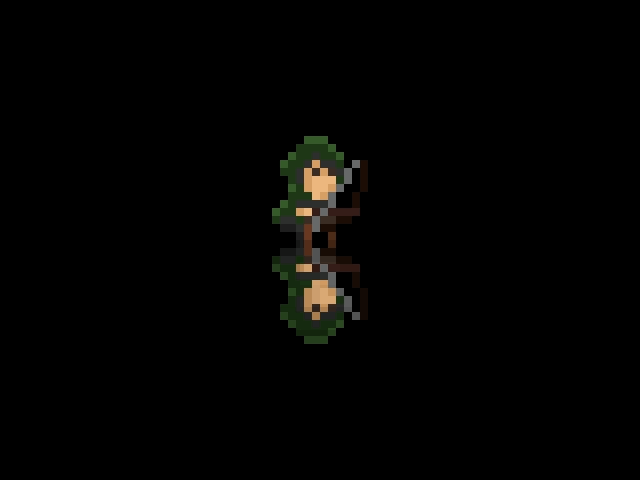Alternatively, here’s a square map: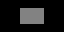In this case I’ve also added a blue background to highlight the reflecting area: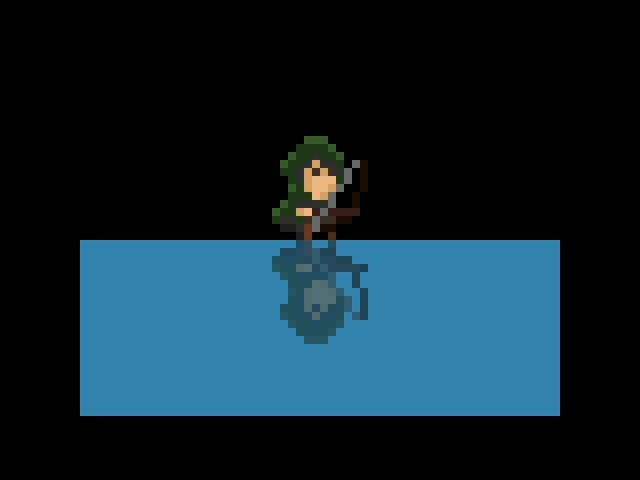That’s all folks.

PS: I was about to call it good at that point, when I noticed that this technique works equally well to generate shadows for sprites. I’m not quite sure if I will use it in my engine, but here’s an example of what a scene with dynamically generated shadows can look like: